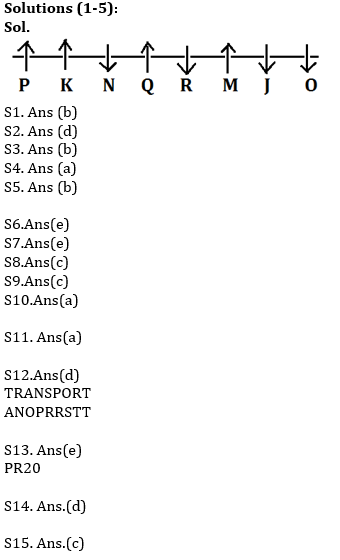Latest Banking jobs   »   Reasoning Ability Quiz For ESIC- UDC,...

# Reasoning Ability Quiz For ESIC- UDC, Steno, MTS Prelims 2022- 27th January

Directions (1-5): Study the following information carefully and answer the question given below:
M, N, O, P, Q, R, J and K are sitting in a straight line but not necessarily in the same order. Some of them are facing south while some are facing north. Only two people sit to the right of M. N sits third to the left of M. Only one person sits between N and R. R sits to the immediate right of Q. Only one person sits between Q and K. Both the immediate neighbours of N face the same direction. M faces north. O sits third to the left of R. N faces the opposite direction of M. J does not sit at any of the extremes ends of the line. P faces the same direction as Q. Both J and O face the opposite direction of K.

Q1. How many persons in the given arrangement are facing North?
(a) More than four
(b) Four
(c) One
(d) Three
(e) Two

Q2. Four of the following five are alike in a certain way, and so form a group. Which of the following does not belong to the group?
(a) Q, R
(b) K, J
(c) N, M
(d) N, J
(e) P, O

Q3. What is the position of R with respect to K?
(a) Second to the left
(b) Third to the right
(c) Third to the left
(d) Fifth to the right
(e) Second to the right

Q4. Who amongst the following sits exactly between K and Q?
(a) N
(b) J
(c) R
(d) Q
(e) O

Q5. Who is sitting 2nd to the right of N?
(a) K
(b) P
(c) R
(d) Q
(e) None of these.

Directions (6-10): Following questions are based on the five three-digit numbers given below.

947 376 694 739 863

Q6. If all the digits in each of the numbers are arranged in increasing order within the number then, which of the following number will become the lowest in the new arrangement of numbers?
(a) 947
(b) 863
(c) 739
(d) 694
(e) 376

Q7. If all the numbers are arranged in ascending order from left to right then, which of the following will be the sum of all the three digits of the number which is 2nd from the right in the new arrangement?
(a) 18
(b) 19
(c) 15
(d) 16
(e) None of these

Q8. What will be the difference when third digit of the 3rd lowest number is multiplied with the second digit of the highest number and third digit of the 2nd highest number is multiplied with the second digit of the lowest number?
(a) 21
(b) 20
(c) 15
(d)16
(e) None of these

Q9. If the positions of the second and the third digits of each of the numbers are interchanged then, how many even numbers will be formed?
(a) None
(b) One
(c) Two
(d) Three
(e) Four

Q10. If one is added to the second digit of each of the numbers and one is subtracted from the third digit of each number then, how many numbers thus formed will be divisible by three in new arrangement?
(a) None
(b) One
(c) Two
(d) Three
(e) Four

Q11. If in the number 639429687, 1 is added to each of the digit which is less than five and 1 is subtracted from each of the digit which is greater than five then how many digits are repeating in the number thus formed?
(a) Two
(b) One
(c) None
(d) Three
(e) Four

Q12. The position of how many alphabets will remain unchanged if each of the alphabets in the word ‘TRANSPORT’ is arranged in alphabetical order from left to right?
(a) Four
(b) Three
(c) None
(d) One
(e) Two

Q13. Which of the following elements should come in a place ‘?’ ?
DF8 HJ12 LN16 ?
(a) PR19
(b) PR18
(c) PR21
(d) PR22
(e) None of these

Q14. Which of the following symbols should replace the sign (@) and (%) respectively in the given expression in order to make the expression B ≥ C and H > K definitely true?
B ≥ D ≥ F = E = K @ C ≤ A % H
(a) ≤, =
(b) ≤, ≤
(c) >, ≤
(d) =, <
(e) ≥, <

Q15. Which of the following will be definitely true if the given expression A≥D≥G=K<H=M<Q≤R is definitely true?
(a) A < H
(b) D > G
(c) R > K
(d) R ≥ G
(e) A < M

Solutions#### Congratulations!